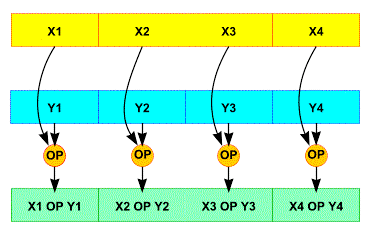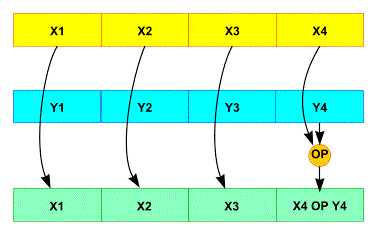# Category: Sse tutorial

## SSE usage Tutorial

Hi all,

SSE usage is a bit tricky

You have to see see registers as vectorial not a linear and their size is depending of the context.

For instance xmm0 (a SSE 128 bytes register)can be seen as 2*64 bits register or 4*32 bits register or 8*16  bits register or 16*8 bits register.

## So what is the aim of this ?

If you want to add two arrays the algorithm wil be

```int x  ; //let's say that sizeof(int) =32
int y;
int z;

c=ab
c=a+b
c=a+b
c=a+b```

In assembler it gives :

```       .loc 1 6 0
mov     edx, DWORD PTR [rbp-48]
mov     eax, DWORD PTR [rbp-32]
mov     DWORD PTR [rbp-16], eax
.loc 1 7 0
mov     edx, DWORD PTR [rbp-44]
mov     eax, DWORD PTR [rbp-28]
mov     DWORD PTR [rbp-12], eax
.loc 1 8 0
mov     edx, DWORD PTR [rbp-40]
mov     eax, DWORD PTR [rbp-24]
mov     DWORD PTR [rbp-8], eax
.loc 1 9 0
mov     edx, DWORD PTR [rbp-36]
mov     eax, DWORD PTR [rbp-20]
mov     DWORD PTR [rbp-4], eax
mov     eax, 0```

For instance c = a+b is generated like that:

```       .loc 1 6 0
mov     edx, DWORD PTR [rbp-48] ; a
mov     eax, DWORD PTR [rbp-32] ; b
add     eax, edx ;eax <- a+b
mov     DWORD PTR [rbp-16], eax ; c = eaz
```

And we are doing that 4 times. But thanks to the SSE extension operator we can do it with less instructions

The Streaming SIMD Extensions enhance the x86 architecture in four ways:

1. 8 new 128-bit SIMD floating-point registers that can be directly addressed;
2. 50 new instructions that work on packed floating-point data;
3. 8 new instructions designed tocontrol cacheability of all MMX and 32-bit x86 data types, including the ability to stream data to memory without polluting the caches, and to prefetch data before it is actually used;
4. 12 new instructions that extend the instruction set.

This set enables the programmer to develop algorithms that can mix packed, single-precision, floating-point and integer using both SSE and MMX instructions respectively.

Intel SSE provides eight 128-bit general-purpose registers, each of which can be directly addressed using the register names XMM0 to XMM7. Each register consists of four 32-bit single precision, floating-point numbers, numbered 0 through 3.

SSE instructions operate on either all or the least significant pairs of packed data operands in parallel. The packed instructions (with PS suffix) operate on a pair of operands, while scalar instructions (with SS suffix) always operate on the least significant pair of the two operands; for scalar operations, the three upper components from the first operand are passed through to the destination.

### There are two ways to use SSE registers

#### Scalar the same 4 instructions on 4 datas#### Packed(thanks to Stefano Tommesani)

So let’s return to our code. I think you gonna understand where I want to go. I we fill two registers with 4 values (a..a) in one register and (c..c), add them together and put the result in a third register. With this solution we will do only one addition.

```#include
#include
#include

void p128_hex_u8(__m128i in) {
uint8_t v;
_mm_store_si128((__m128i*)v, in);
printf("v16_u8: %x %x %x %x | %x %x %x %x | %x %x %x %x | %x %x %x %xn",
v, v,  v,  v,  v,  v,  v,  v,
v, v, v, v, v, v, v, v);
}

void p128_hex_u16(__m128i in) {
uint16_t v;
_mm_store_si128((__m128i*)v, in);
printf("v8_u16: %x %x %x %x,  %x %x %x %xn", v, v, v, v, v, v, v, v);
}

void p128_hex_u32(__m128i in) {
uint32_t v __attribute__((aligned (16)));
_mm_store_si128((__m128i*)v, in);
printf("v4_u32: %x %x %x %xn", v, v, v, v);
}

void p128_dec_u32(__m128i in) {
uint32_t v __attribute__((aligned (16)));
_mm_store_si128((__m128i*)v, in);
printf("v4_u32: %d %d %d %dn",(uint32_t) v, (uint32_t) v, (uint32_t)v,(uint32_t) v);
}

void p128_hex_u64(__m128i in) {
long long v;  // uint64_t might give format-string warnings with %llx; it's just long in some ABIs
_mm_store_si128((__m128i*)v, in);
printf("v2_u64: %llx %llxn", v, v);
}

int main(){
uint32_t a  ={1,2,3,4}; //let's say that sizeof(int) = 32
uint32_t b = {11,12,13,14};
uint32_t c;

c=a+b;
c=a+b;
c=a+b;
c=a+b;
printf("Result %d %d %d %dn",c,c,c,c);

__m128i a1 = _mm_set_epi32(a, a, a, a);
__m128i b1 = _mm_set_epi32(b, b, b, b);